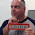## Wednesday, September 22, 2021

### New BiF added to SORTA to make sorting of data structure arrays easierI use data structure arrays in many of my RPG programs. It has always been impossible to sort the data in the data structure array by more than subfield, that is until the latest Technology Refreshes, IBM i 7.4 TR5 and 7.3 TR11.

Included within the new TRs is a Built in Function, BiF, for RPG's Sort Array operation code, SORTA, that allows me to sort by more than one data structure array subfield. The syntax for using %FIELDS with a data structure array is:

 ```SORTA array-name %FIELDS(subfield-1 : subfield-2 : subfield-3) ; SORTA(D) array-name %FIELDS(subfield-1 : subfield-2 : subfield-3) ; ```

The first example statement will sort the subfields in ascending order. As the second statement has the (D) it will sort in descending order.

• array-name:  Name of the data structure array
• subfield-1:  Name of the first data structure subfield to sort by. Multiples can be entered, separated by the colon symbol ( : )

Without further ado I am going to jump straight into my example program.

 ```01 **free 02 dcl-ds Array qualified dim(5) ; 03 Name char(15) ; 04 City char(15) ; 05 Score packed(5:2) ; 06 end-ds ; ```

Line 1: It is 2021 so my code is totally free RPG.

Lines 2 – 6: The definition of my data structure array. It has three subfields. The array has five elements maximum.

I am not going to show how I loaded data structure array, as I think it is a waste of space in this post. The array contains the following:

 ```ARRAY.NAME(1) = 'JANE ' ARRAY.CITY(1) = 'LOS ANGELES ' ARRAY.SCORE(1) = 075.10 ARRAY.NAME(2) = 'JANE ' ARRAY.CITY(2) = 'TUCSON ' ARRAY.SCORE(2) = 056.70 ARRAY.NAME(3) = 'ALBERT ' ARRAY.CITY(3) = 'TUCSON ' ARRAY.SCORE(3) = 022.20 ARRAY.NAME(4) = 'WILFREDA ' ARRAY.CITY(4) = 'SANTA FE ' ARRAY.SCORE(4) = 075.10 ARRAY.NAME(5) = 'ANGELA ' ARRAY.CITY(5) = 'OMAHA ' ARRAY.SCORE(5) = 044.50 ```

Things to notice are:

1. There are two entries with the name Jane. One in Los Angeles, the other in Tucson.
2. There are two entries from the city of Tucson, Albert and Jane.

Before the new TRs if I wanted to sort the data structure array I could only do so by a single subfield:

 ```07 sorta Array(*).Name ; ```

Which gives me:

 ```ARRAY.NAME(1) = 'ALBERT ' ARRAY.CITY(1) = 'TUCSON ' ARRAY.SCORE(1) = 022.20 ARRAY.NAME(2) = 'ANGELA ' ARRAY.CITY(2) = 'OMAHA ' ARRAY.SCORE(2) = 044.50 ARRAY.NAME(3) = 'JANE ' ARRAY.CITY(3) = 'TUCSON ' ARRAY.SCORE(3) = 056.70 ARRAY.NAME(4) = 'JANE ' ARRAY.CITY(4) = 'LOS ANGELES ' ARRAY.SCORE(4) = 075.10 ARRAY.NAME(5) = 'WILFREDA ' ARRAY.CITY(5) = 'SANTA FE ' ARRAY.SCORE(5) = 075.10 ```

If I wanted to sort by the City and then Name I could not have done it before. Now, thanks to the new BiF, I can:

 ```08 sorta Array %fields(City : Name) ; ```

This gives me:

 ```ARRAY.NAME(1) = 'JANE ' ARRAY.CITY(1) = 'LOS ANGELES ' ARRAY.SCORE(1) = 075.10 ARRAY.NAME(2) = 'ANGELA ' ARRAY.CITY(2) = 'OMAHA ' ARRAY.SCORE(2) = 044.50 ARRAY.NAME(3) = 'WILFREDA ' ARRAY.CITY(3) = 'SANTA FE ' ARRAY.SCORE(3) = 075.10 ARRAY.NAME(4) = 'ALBERT ' ARRAY.CITY(4) = 'TUCSON ' ARRAY.SCORE(4) = 022.20 ARRAY.NAME(5) = 'JANE ' ARRAY.CITY(5) = 'TUCSON ' ARRAY.SCORE(5) = 056.70 ```

Notice how Jane from Los Angeles come first, as "Los Angeles" is the lowest value City.

"Tucson" is the highest value city so its entries appear at the end of the array. "Albert" is a lower value so it comes before "Jane".

In this example I am going to sort the array in descending order so the highest score will come first, then I am sorting by City and Name.

 ```09 sorta(d) Array %fields(Score : City : Name) ; ```

The contents of the array look like:

 ```ARRAY.NAME(1) = 'WILFREDA ' ARRAY.CITY(1) = 'SANTA FE ' ARRAY.SCORE(1) = 075.10 ARRAY.NAME(2) = 'JANE ' ARRAY.CITY(2) = 'LOS ANGELES ' ARRAY.SCORE(2) = 075.10 ARRAY.NAME(3) = 'JANE ' ARRAY.CITY(3) = 'TUCSON ' ARRAY.SCORE(3) = 056.70 ARRAY.NAME(4) = 'ANGELA ' ARRAY.CITY(4) = 'OMAHA ' ARRAY.SCORE(4) = 044.50 ARRAY.NAME(5) = 'ALBERT ' ARRAY.CITY(5) = 'TUCSON ' ARRAY.SCORE(5) = 022.20 ```

Notice that Wilfreda and Jane of Los Angeles have the same score. Wilfreda comes first as sorting in descending order her name comes before Jane's.

I can use the Substring Array BiF, %SUBARR, with the %FIELDS BiF. For example I only want to sort the first three elements of the array I can use the following:

 ```10 sorta %subarr(Array : 1 : 3) %fields(Name : City) ; ```

And I now have:

 ```ARRAY.NAME(1) = 'JANE ' ARRAY.CITY(1) = 'LOS ANGELES ' ARRAY.SCORE(1) = 075.10 ARRAY.NAME(2) = 'JANE ' ARRAY.CITY(2) = 'TUCSON ' ARRAY.SCORE(2) = 056.70 ARRAY.NAME(3) = 'WILFREDA ' ARRAY.CITY(3) = 'SANTA FE ' ARRAY.SCORE(3) = 075.10 ARRAY.NAME(4) = 'ANGELA ' ARRAY.CITY(4) = 'OMAHA ' ARRAY.SCORE(4) = 044.50 ARRAY.NAME(5) = 'ALBERT ' ARRAY.CITY(5) = 'TUCSON ' ARRAY.SCORE(5) = 022.20 ```

Jane of Los Angeles who was in third place is now first, and Wilfreda is now third. Fourth and fifth places remain unchanged.

I know I am going to be using this new BiF a lot in many of my programs. It is going to make my processing of the data in data structure arrays so much easier to do.

You can learn more about the %FIELDS Built in Function used with RPG's Sort Array operation code from the IBM website here.

This article was written for IBM i 7.4 TR5 and 7.3 TR11.

1.Good BiF SortA thanks for sharing

2.Outstanding Knowledge Transfer Thanks Sir 🙂

3.Great tip. Thanks.

4.Does it work for multioccurance data structure?

1.No.

In modern RPG you should not be using MODS. Data Structure arrays are what you should be using.

5.1.6.Reynaldo Dandreb MedillaMarch 12, 2023 at 8:44 AM

thanks for sharing Simon, a nice array sorting feature

To prevent "comment spam" all comments are moderated.
```